Register for Maths, Science, English, Reasoning Olympiad Exams Register here | Book Free Demo for Live Olympiad Classes here | Check Olympiad Exam Dates here | Buy Practice Papers for IMO, IOM, HEO, IOEL etc here | Login here to participate in all India free mock test on every Saturday

# Geometry and Shapes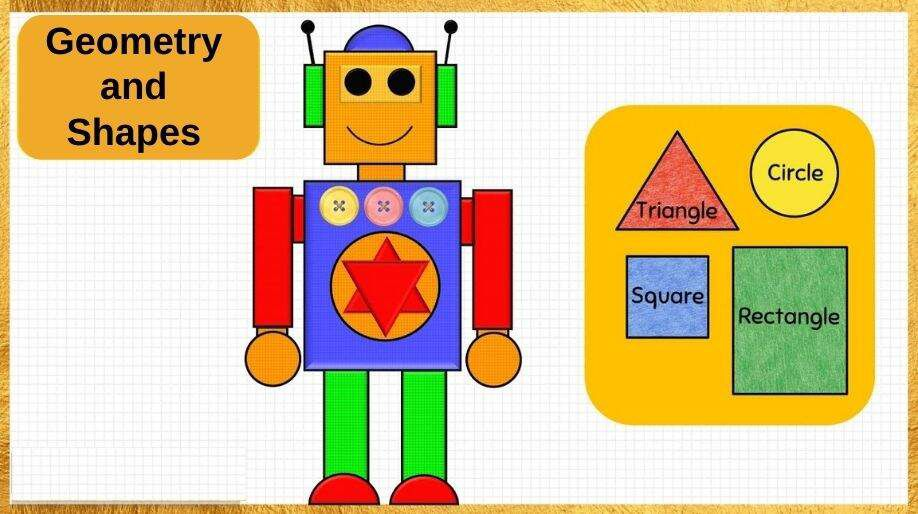Geometry and Shapes

Geometry is useful in recognizing the shapes. Do you want to know the shape of` your house, toys and favorite chocolate?

Let Us Revise Few Shapes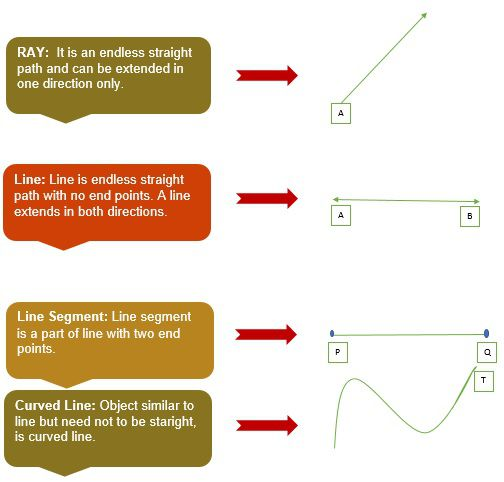Now we will study the shapes formed with the help of sleeping lines, slanting lines, curved lines!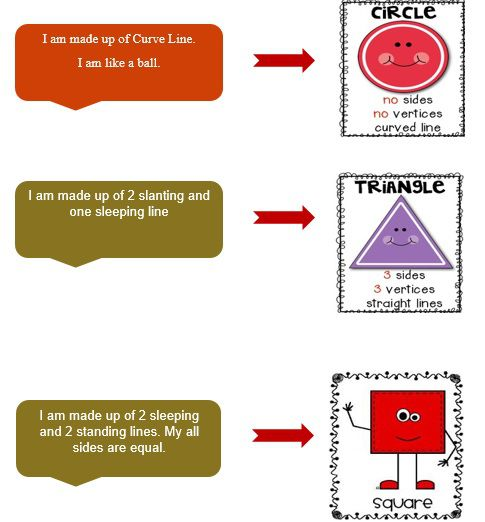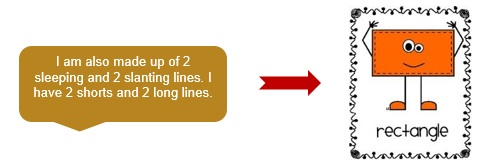Angles

Angle is the figure formed by two rays, called sides of angle. Both sides have common end point, called vertex of the angle.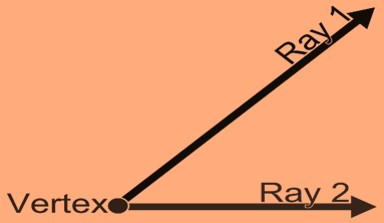• A positive angle goes anti-clockwise.
• A negative angle goes clockwise.

Type of Angles

 Sr No Angle Type Description 1 Acute Angle An angle less than 90° 2 Right Angle An angle that is equal to 90° 3 Obtuse Angle An angle greater than 90° but less than 180° 4 Straight Angle An angle equals to 180° 5 Reflex Angle An angle greater than 180°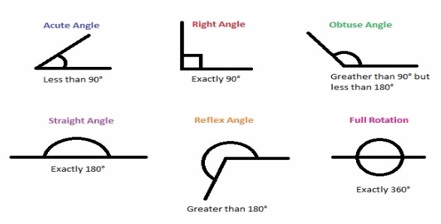Circles

A circle is the locus of all the points that are equidistant from central point.

• Radius of Circle: It is the distance from the center to the edge.

• Diameter: It starts from one end of the circle and ends on another while going through center.

• Circumference: It is the distance around the edge of circle.

• Area of Circle: The area of circle is Π times the radius squared.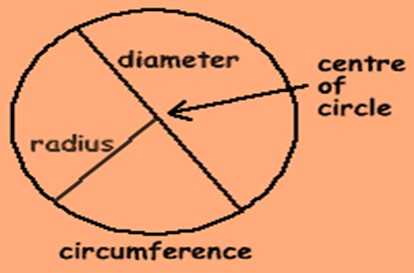Example 1: A circle has radius 50 cm. Calculate its circumference.

Sol: Diameter = 2×50 = 100 cm

Circumference = Π×100 = 314 cm

Example 2: Find the area of circle having radius 2 m?

Sol: Area = Π×R² = 3.14×2×2 = 3.14×4 = 12.56 m²

Practice These Questions

• Find out the number of sleeping lines, slanting lines, curved lines, circles, square, triangle and rectangle in the figure below: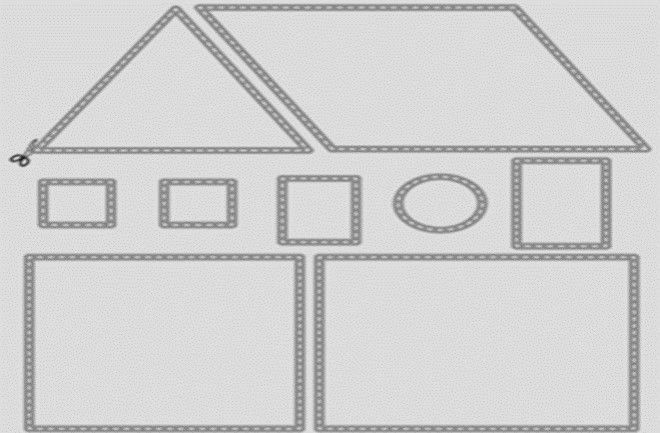• Name the angles:
1. 78°
2. 156°
• 45°
1. 255°
2. 180°

• Find out the diameter, circumference and area of circle whose radius is 20 cm?

Recap

• Ray is extended to one end only.
• Line is extended towards both ends.
• Line segment is a part of line with two end points.
• Angle less than 90° is acute angle; angle more than 90° is obtuse angle.
• 90°angle is called right angle.
• Radius of circle is half of its diameter.

## Quiz for Geometry and Shapes

 Q.1 Which of the following is a closed figure? a)b)c)d)Q.2 Name the vertex in the given angle.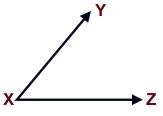a) X b) Y c) XY d) XZ
 Q.3 What will be the area of a rectangle of dimensions 15 m and 20 m? a) 35 sq. m b) 225 sq. m c) 300 sq. m d) 400 sq. m
 Q.4 Which of the following has a fixed length? a) Point b) Line c) Ray d) Line segment
 Q.5 If the area of a square is 64 square cm. What is the side of the square? a) 32 cm b) 8 cm c) 16 cm d) 14 cm
 Q.6 Suhani wants to make a rectangular wall hanging such that perimeter of its boundary should 45 cm, keeping length of rectangle 12 cm. What will be the breadth of the wall hanging? a) 21 cm b) 33 cm c) 15 cm d) 10.5 cm
 Q.7 A garden is 50 m long and 45 m broad. How much area does it constitute? a) 100 sq. m b) 90 sq. m c) 2250 sq. m d) 2000 sq. m
 Q.8 If radius of a circle is 7 cm. What will the length of its longest chord? a) 3.5 cm b) 14 cm c) 7 cm d) 21 cm
 Q.9 Sohan's mother has a bangle of radius 3 cm. Find the diameter of the bangle. a) 6 cm b) 1.5 cm c) 12 cm d) 9 cm
 Q.10 Divya made a poster of the following shape with each side equals to 16 cm. She needs coloured tape to cover the boundary. What length of the tape will be needed?a) 256 cm b) 64 cm c) 80 cm d) 48 cm